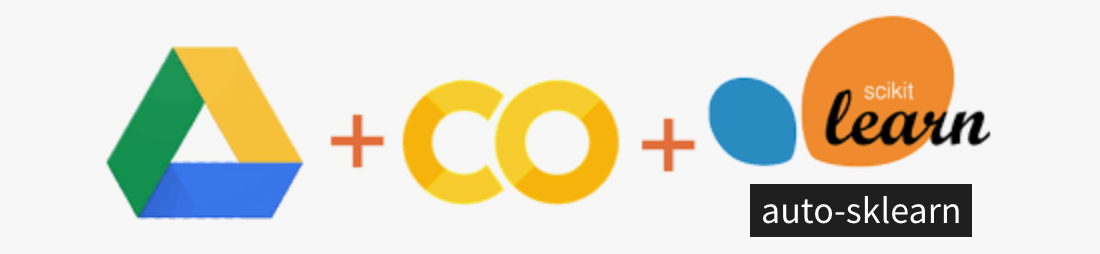# Google Colab and AutoML: Auto-sklearn SetupLogos of Google Drive + Colab + Scikit-learn + Auto-sklearn
`!sudo apt-get install build-essential swig!pip install auto-sklearn==0.11.1`
`import autosklearn.regressionautoml = autosklearn.regression.AutoSklearnRegressor(  time_left_for_this_task=120,  per_run_time_limit=30,  n_jobs=1)automl.fit(  X_train_transformed,  y_train)`
`import sklearn.metricspredictions = automl.predict(X_test_transformed)sklearn.metrics.r2_score(y_test, predictions)# output: 0.1862`
`from sklearn.ensemble import RandomForestRegressorfrom sklearn.model_selection import GridSearchCVmodel = RandomForestRegressor(max_depth=3, random_state=0)parameters = {  "max_depth": (2, 3, 5)}grid = GridSearchCV(model, parameters, cv=5, scoring="r2")grid.fit(X_train_transformed, y_train.values.ravel())`
`predictions = grid.predict(X_test_transformed)sklearn.metrics.r2_score(y_test, predictions)# output: 0.0982`